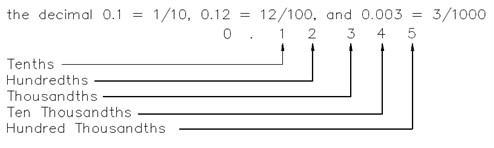## Decimals Assignment Help

Assignment Help: >> Introductory Mathematics - Decimals

Decimals:

Chapter Decimals covers the processes of addition, subtraction, multiplication & division of numbers in decimal form.

APPLY one of the arithmetic operations of addition, subtraction, multiplication & division of fractions through conversion to decimal form using a calculator.

APPLY one of the arithmetic operations of addition, subtraction, multiplication & division using decimals.

While using numbers, the operator will use whole numbers at times and decimal numbers at other times. A decimal number is a number which is given in decimal form, such as 15.25.  The decimal portion is equivalent to a certain "fraction-of-one," thus allowing values among integer numbers to be expressed.

A decimal is a linear array of integers which represents a fraction.  Every decimal place implies a multiple of a power of 10.

Example:Addition and Subtraction of Decimals Decimal to Fraction Conversion Dividing Decimals Fraction to Decimal Conversion Multiplying Decimals Rounding Off